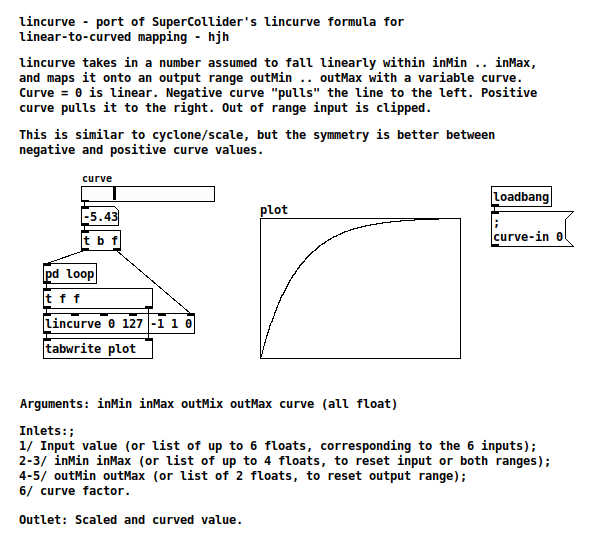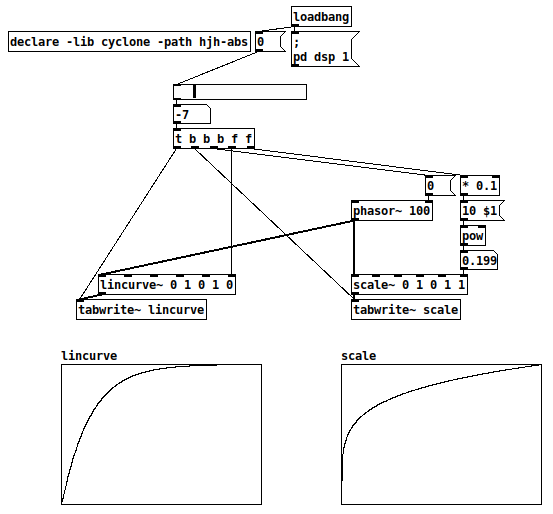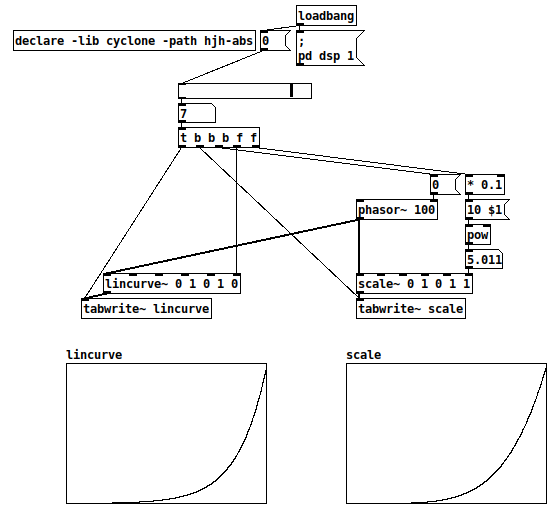Here's a Pd-vanilla version of a linear-to-curved range mapping formula from SuperCollider. Published at https://github.com/jamshark70/hjh-abs . There's a message-rate and signal-rate version.(Helpfile typo has been fixed but I won't bother to upload a new screenshot.)

This is, of course, close to cyclone's [scale]. It's close -- the symmetry is different (help file says "better" but actually that's a matter of taste, or situational need). For reciprocal values of [scale]'s "exponential" factor, the respective curves seem to be a reflection around the diagonal (x <--> y); for negated values of [lincurve]'s curve factor, one curve is a 180-degree rotation of the other (x --> -x and y --> -y). Compare -7 vs +7 lincurve against 0.2 vs 5.0 scale:

-7 curve, ~0.2 exp:+7 curve, ~5.0 exp (lincurve and scale are very very close here!):hjh